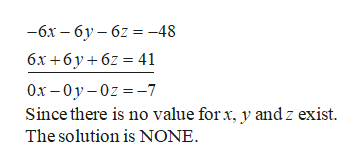# Solve. (Enter your answers as a comma-separated list. If there are infinitely many solutions, enter a parametric solution. If there is no solution, enter NONE.)2x + 2y + 2z = 166x + 6y + 6z = 413x + 3y + 4z = 31(x, y, z) =  Solve the following system of equations. (Enter your answers as a comma-separated list. If there are infinitely many solutions, enter a parametric solution using t and/or s. If there is no solution, enter NONE.)x1 − 2x2 + x3 = −12x1 + x2 − 2x3 = 1x1 + 3x2 − 3x3 = 2(x1, x2, x3) =

Question
73 views

Solve. (Enter your answers as a comma-separated list. If there are infinitely many solutions, enter a parametric solution. If there is no solution, enter NONE.)

 2x + 2y + 2z = 16 6x + 6y + 6z = 41 3x + 3y + 4z = 31

(x, y, z) =

Solve the following system of equations. (Enter your answers as a comma-separated list. If there are infinitely many solutions, enter a parametric solution using t and/or s. If there is no solution, enter NONE.)

 x1 − 2x2 + x3 = −1 2x1 + x2 − 2x3 = 1 x1 + 3x2 − 3x3 = 2
(x1, x2, x3) =
check_circle

Step 1
1. Consider the equations as follows.
Step 2

Multiply equation (1) by –3 and add with equation (1).help_outlineImage Transcriptionclose—бх — бу—62 %3D -48 бх +6у+62 %3 41 Ох — 0у-0z 3-7 Since there is no value for x, y and z exist The solution is NONE. fullscreen
Step 3
1. Consider the equations as foll...

### Want to see the full answer?

See Solution

#### Want to see this answer and more?

Solutions are written by subject experts who are available 24/7. Questions are typically answered within 1 hour.*

See Solution
*Response times may vary by subject and question.
Tagged in

### Equations and In-equations